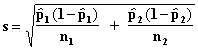LEGACY CONTENT. If you are looking for Voteview.com, PLEASE CLICK HERE

This site is an archived version of Voteview.com archived from University of Georgia on May 23, 2017. This point-in-time capture includes all files publicly linked on Voteview.com at that time. We provide access to this content as a service to ensure that past users of Voteview.com have access to historical files. This content will remain online until at least January 1st, 2018. UCLA provides no warranty or guarantee of access to these files.

### 45-733 PROBABILITY AND STATISTICS I

A SHORT GUIDE TO CONFIDENCE INTERVALS

```
Confidence        Sample Size           Variance          What Test
Interval     (Type of Distribution)                         to Use

s2 known            IA Z
Small Sample (Normal)
s2 unknown          IIIA t
m

s2 known            IA Z
Large Sample (Any)
s2 unknown          IIA Z

s2 known            IB Z
Small Sample (Normal)
s2 unknown          IIIB t
m1 - m2
s2 known            IB Z
Large Sample (Any)
s2 unknown          IIB Z

p         Large Sample                NA                  VA Z

p1 - p2       Large Sample                NA                  VB Z

s2         Small and Large (Normal)    NA                  IV c2
```

1. Confidence Intervals for m When s2 is known

These tests are used when the random sample is drawn from a Normal distribution with a known variance.

1. Confidence Interval for m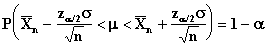Confidence Limits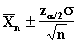2. Confidence Interval for the Difference Between Two Means

In this sampling situation the variances of the two populations are known.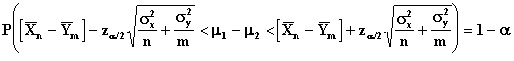Confidence Limits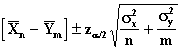2. Large Sample Confidence Intervals for m (n ³ 30)

When the sample size is large, the Central Limit Theorem allows us to calculate confidence intervals for any distribution using these formulas (compare these with the ones for proportions in V).

1. Confidence Interval for m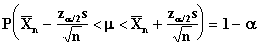where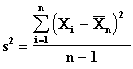Confidence Limits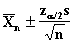2. Confidence Interval for the Difference Between Two Means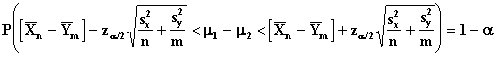Confidence Limits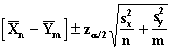3. Small Sample Confidence Intervals for m (n £ 29)

These tests are used when the random sample is drawn from a Normal distribution with unknown variance.

1. Confidence Interval for m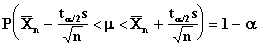whereConfidence Limits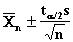2. Confidence Interval for the Difference Between Two Means

In this sampling situation the variances of the two populations are assumed to be the same.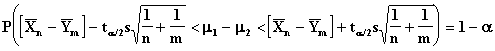where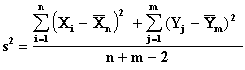4. Confidence Interval For s2

This test is used when the random sample is drawn from a Normal Distribution.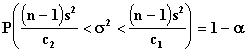whereConfidence Limits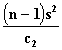and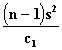5. Large Sample Confidence Intervals For Proportions

When the sample size is large, the Central Limit Theorem allows us to calculate confidence intervals for proportions (technically the random sample is drawn from a Bernoulli distribution). Recall that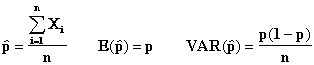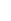Using the Central Limit Theorem we assume that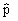~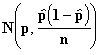1. Confidence Interval for p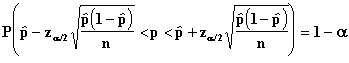Confidence Limits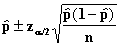2. Confidence Interval For the Difference Between Two Proportions

Let p1 and p2 be the two population proportions and let n and m be the sample sizes from the two populations respectively. Using the Central Limit Theorem we assume that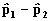~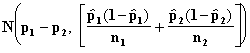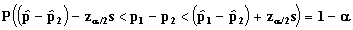where